Optics
Volume 4, Issue 3-1, June 2015, Pages: 18-23

Calibration of a Fringe Projection 3D Measurement System Using an Equi-Phase Coordinate Method Based on Two-Reference-Plane

Dai Meiling, Yang Fujun, He Xiaoyuan

Department of Engineering Mechanics, Southeast University, Nanjing, PR China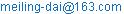Dai Meiling, Yang Fujun, He Xiaoyuan. Calibration of a Fringe Projection 3D Measurement System Using an Equi-Phase Coordinate Method Based on Two-Reference-Plane. Optics. Special Issue: Optical Techniques for Deformation, Structure and Shape Evaluation. Vol. 4, No. 3-1, 2015, pp. 18-23. doi: 10.11648/j.optics.s.2015040301.15

Abstract: Calibration is to transform the 2D phase information to the world coordinates in a fringe projection 3D measurement system. For the phase-to-height conversion, an equi-phase coordinate method based on two-reference-plane is proposed in this paper. The surface height is calculated by a linear interpolation using the coordinates where have the identical phase value of the object and the two reference planes. The conventional method, called equi-coordinate phase method in this paper, builds the function of the absolute phase and height by using the absolute phase obtained by subtracting the phase of object from that of the reference plane in the same coordinate. The proposed method can handle phase-to-height conversion and non-sinusoidal error caused by nonlinear response of the fringe projection system in one go. Theoretical and experimental analysis is given to prove the validity of the proposed method. Result indicates that the RMS error produced by equi-phase coordinate method is less half of equi-coordinate phase method when the primary error source is from the non-sinusoidal fringe patterns.

Keywords: Three-Dimensional Shape Measurement, Calibration, Equi-Phase Coordinates, Non-Sinusoidal Error

1. Introduction

Increasing demands in military, industrial and public entertaining applications have driven the research and development of optical techniques for shape measurement. The structured light projection technique  is one of the most widely adopted, owing to its simplicity in principle and relative ease of implementation. The projected light pattern may be in a variety of forms, from black-and-white bar code , multiple grey-scale strips , to continuous sine wave fringe patterns [2, 3, 4]. The regional two-dimensional (2D) shift of a pattern in the recorded image(s) owing to surface height variation can be extracted by a data-processing algorithm. The 2D distance in pixel is then converted to the surface height of the object.

Triangulation and system geometrical arrangement lie in the core of the conversion, for which a calibration process is often needed [5-15]. In terms of strategy, the calibration may be realized by either retrieving system geometrical parameters directly [6, 10, 11, 14] or extracting a few coefficients, sufficient to describe the equation of conversion [5, 7, 8, 9, 12, 13, 15]. The former is perhaps preferable if the measurement targets are varying in size because given a full set of system parameters, including projection and imaging geometry, intensity non-uniformity, lens distortion, etc, measurement can be performed on objects of arbitrary size theoretically. In practice, it is difficult to retrieve the full set of system parameters. Often, it is only feasible to retrieve a subset of such parameters upto certain accuracy. Primarily due to this reason, the latter strategy is preferable for targets of similar dimension or for a once-only shape measurement task – it is worthwhile to perform a dedicated calibration process if the measurement accuracy is important.

Dedicated system calibration is especially necessary for a group of structured light methods - fringe projection profilometry [2-4] because they have stringent requirement on the sinusoidal intensity distribution in order to achieve high precision. Conventionally, the two important issues in fringe projection system calibration: phase-to-height conversion [5, 7-13, 15] and non-sinusoidal fringe pattern due to intensity distortion [16-19], were handled separately. The second problem was tackled by pre-adjusting the projection intensity to compensate distortion [17, 18] or by post-filtering algorithms to remove the error . Both are effective in some situations; however, pre-adjusting the projector requires an estimation of the intensity distortion, which adds an additional layer of complexity; post-filtering the images removes surface profile features at a frequency similar to the error (Such features are commonly found in mechanical parts manufactured by the lathe, miller, grinder, etc.).

In this paper, an equi-phase coordinate method based on two-reference-plane was proposed, and that is able to handle phase-to-height conversion and intensity distortion in one go. In the following sections, the principle of our method is developed and the validity of the proposed calibration method is proved based on experimental results.

2. Phase-to-Height Conversion

Our phase-to-height conversion method is shown in Fig.1: two parallel reference planes, a projector for fringe projection and a camera for deformed fringe recording. The distance, z2z1=h, between the two planes is artificially introduced and precisely measured.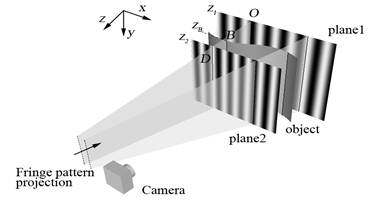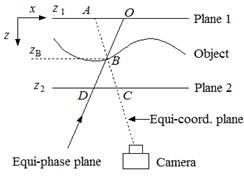(a)                                                        (b)

Fig. 1. Diagram of the two-plane fringe pattern projection method: (a) 3D calibration system; (b) top view of (a).

When the intensity of a sinusoidal fringe with frequency f is distributed along the x-axis, the phase value of the fringe is monotonically increasing along the x-axis. For a fixed x coordinate, the phase is a constant along the y-axis. The projection plane that hosts identical phase value may be called an equi-phase plane, which is projected onto the plane 1, plane 2 and object with the intersection O, D, B respectively (Fig.1(b)). Accordingly, the plane that has the same image coordinate may be called an equi-coordinate plane, which is projected onto the plane 1, plane 2 and object with the intersection A, C, B respectively (Fig.1(b)). From similar triangles, OAB and DCB: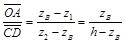(1)

Where z1 and z2 are the z coordinates of the plane 1 and plane 2 points illuminated by the equi-phase plane, respectively. zB is the z coordinates of the object surface point illuminated by the same equi-phase plane.

Since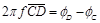and, it can be obtained: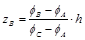(2)

Where ϕA, ϕB , ϕC, ϕD and ϕO are the projected fringe phase angle corresponding to points A, B, C , D and O, respectively. In Eq.(2), ϕA, ϕB , ϕC are different, but have the same image coordinate. This method may be called the equi-coordinate phase method, which is conventionally used for phase-to-height conversion.

Since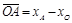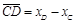, and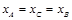, which are substituted into Eq.(1) , here, we propose an equi-phase coordinate method based on two-reference-plane as below: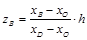(3)

Where xA, xB , xC, xD and xO are the x coordinates of points A, B, C , D and O, respectively. For a point xB of object surface with a phase ϕ, we can find the identical phase value coordinates xO , xD on the reference plane 1, plane 2 respectively. xO or xD usually does not fall on a pixel but in between two pixels. Linear interpolation is applied to obtain the sub-pixel x coordinate. So in Eq. (3), xO and xD are of sub-pixel precision and xB is an integer.

If the recorded fringe pattern is purely sinusoidal, accurate height values can be calculated by either Eq. (2) or (3). With non-sinusoidal fringe patterns, each phase angle has an additional error component. The error is not random but a high-frequency term added to the original phase angle. Hence, if two phase angles are the same, their error components are theoretically the same as well; practically very close. Since all phase values on an equi-phase plane are affected by the same error, the position of the plane is not shifted or distorted. Consequently, xO, xB, xD in Eq. (3) maintain their original values and the resultant height is relatively unaffected by the non-sinusoidal effect. In contrast, ϕA, ϕB and ϕC in Eq. (2) are different, introducing different error components that cannot be cancelled out; therefore, the calculated height value will be affected by the non-sinusoidal fringe patterns.

The equi-phase coordinate method was originally described in Srinivasan’s 1985 paper . That implementation was given based on one reference plane and required the quantification of several system geometrical parameters. Subsequent improvements of the technique incorporated more than one reference planes but discarded using the equi-phase coordinates in preference to using equi-coordinate points [5, 7, 8, 9, 12, 13, 15]. The latter method is indeed more convenient, since the equi-coordinate points are immediately available. However, as pointed out above and as will be seen from the test results, its accuracy is relatively sensitive to non-sinusoidal fringe patterns caused by intensity distortion.

3. Height-to-World Coordinates Conversion

Based on fringe projection, the height (z world coordinate) of each surface point can be obtained from the previous section. If the camera of the system is prior calibrated, the x and y world coordinates of a surface point can also be obtained from the camera matrix, the height and the image coordinate of the point.

This is supported by the governing equation, expressed in homogeneous coordinates, that maps a 3D world point to a 2D image point :

(xi, yi, 1)T = M· (xw, yw, zw, 1)T     (4)

Where, M is the camera matrix, T denotes matrix transpose, xi and yi are image coordinates, xw, yw and zw are world coordinates (zw is the height). The matrix representation contains three equations, two of which are linearly independent; hence the two unknowns: xw and yw can be calculated.

4. Experiments and Results

In the following experiments, a multi-frequency fringe projection technology combined with 4-step phase-shifting algorithm was used for phase calculation. An Optoma pico pocket projector (PK301) was used to project the fringe patterns. A Point Gray Flea3 Series USB3.0 camera (FL3-U3-32S2C-CS) was used to capture the images. A linear stage (TSA400-BF) was used to shift the reference plane at different positions. Its travel range is 400 mm, and its accuracy is 5 μm. The world coordinate of the system can be arbitrarily chosen: the X and Y axes were set to parallel to the horizontal and vertical edges of a chessboard pattern, respectively (Fig. 2); the Z axis was set to the shifting direction of the linear stage.

First, a calibration chessboard was sequentially placed at three locations with a pure z direction shift of 25mm in between. The first and third locations were treated as reference planes, as shown in Fig. 2. The background images, Figs. 2(a) and 2(c), were used for camera calibration. 36 corner points of the black squares were extracted at subpixel accuracy, based on which the camera matrix in Eq. (4) was obtained .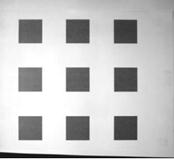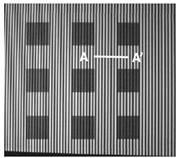(a)                             (b)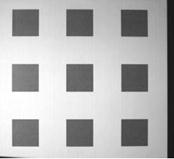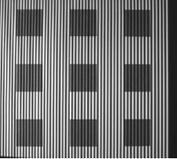(c)                       (d)

Fig. 2. (a),(c) Background image and (b),(d) fringe patterns of the calibration chessboard at the first and third locations.

Table 1. Comparison between the two methods.

 conventional proposed RMS error of the chessboard height measurement at the second location. 0.134mm 0.053mm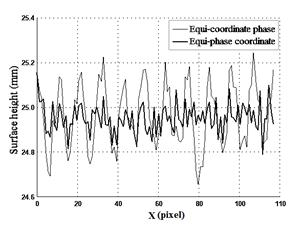Fig. 3. Cross-section A–Alabelled in Fig.2(b) on the chessboard surface obtained by the two methods.

The chessboard surface height at the second location was calculated based on Equations (2) and (3). The region of interest on the chessboard was about 310 by 215mm2, corresponding to 620×430 pixels, and the results were compared as shown in table1, which indicated that the RMS error produced by equi-phase coordinate method (proposed) is almost 40% of the equi-coordinate phase method (conventional). Fig.3 shows a cross-section A–A labelled in Fig.2(b) on the chessboard surface obtained by the two methods. It can be seen that the error amplitude of the equi-phase coordinate method is about one third of the other but the error signal exhibits an even higher frequency. This may be caused by the digitization error in the projector and the camera: if two images are taken sequentially without changing any system components (lighting, projection or imaging equipment), the image data are not identical. Investigation in methods to reduce the digitization error is beyond the scope of this paper; nevertheless, the visibility of such error is an indication that the equi-phase coordinate method has removed the primary error source.

Next, two test objects, as shown in Fig.4, were placed between the same reference planes and surface heights were obtained by the two methods, the world coordinates were obtained using Eq.(4), and then the 3D object surfaces were reconstructed, as shown in Fig.5, in which, the results from the conventional method (Fig.5(a), (b)) exhibited the obvious non-sinusoidal error like stripes. This kind of error was significantly reduced by the proposed method ( Fig.5(c), (d)).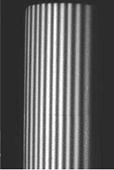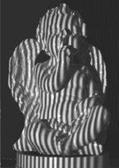(a)             (b)

Fig. 4. Test objects with projected fringe patterns: (a) cylinder and (b) angel model.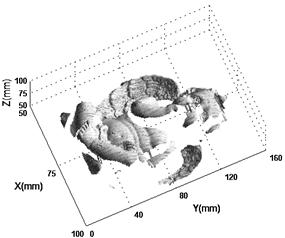(a)                                                     (b)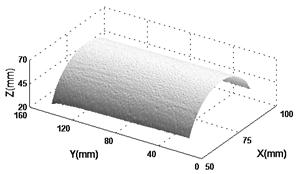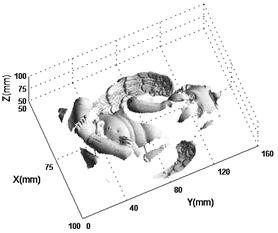(c)                                       (d)

Fig. 5. The reconstruction results of two objects: (a) and (b) results from the conventional method; (c) and (d) results from the proposed method.

5. Conclusion

Base on a two-reference-plane experimental setup, we have demonstrated that the equi-phase coordinate method is able to handle two issues in fringe projection profilometry in one go. They are phase-to-height conversion and error reduction due to non-sinusoidal fringe patterns. Although the history of this approach goes as early as 1985 , the originally described system cannot be conveniently implemented for both tasks. Subsequent improvements of the method have discarded the equi-phase coordinate in preference to the equi-coordinate phase[5, 7, 8, 9, 12, 13, 15]. We have shown analytically and experimentally that the latter suffers from errors due to intensity distortion, which the former is almost immune to.

This research work was supported by the National Science Foundation of China (NSFC) (grants 11272090, 11472081, 11327201, and 11332012).

References

1. J. Geng, "Structured-light 3d surface imaging: a tutorial," Advances in Optics and Photonics,3: 128–160, 2011.
2. M. Takeda and K. Mutoh, "Fourier transform profilometry for the automatic measurement of3-d object shapes," Applied Optics, 22(24): 3977–3982, 1983.
3. V. Srinivasan, H. C. Liu, and M. Halioua, "Automated phase-measuring profilometry of 3-ddiffuse objects," Applied Optics, 23(18): 3105–3108, 1984.
4. S. S. Gorthi and P. Rastogi, "Fringe projection techniques: Whither we are?," Optics andLasers in Engiering, 48(2): 133–140, 2010.
5. W. S. Zhou and X. Y. Su, "A direct mapping algorithm for phase-measuring profilometry,"Journal of Modern Optics, 41(1): 89–94, 1994.
6. D. Q. Huynh, "Calibrating a structured light stripe system: A novel approach," InternationalJournal of Computer Vision, 33(1): 73–86, 1999.
7. G. Sansoni, M. Carocci, and R. Rodella, "Calibration and performance evaluation of a 3-dimaging sensor based on the projection of structured light," IEEE Transactions on Instrumentationand Measurement, 49(3): 628–636, 2000.
8. H. Liu, W. H. Su, K. Reichard, and S. Yin, "Calibration-based phase-shifting projected fringeprofilometry for accurate absolute 3d surface profile measurement," Optics Communications,216: 65–80, 2003.
9. S. Pavageau, R. Dallier, N. Servagent, and T. Bosch, "A new algorithm for large surfacesprofiling by fringe projection," Sensors and Actuators A, 115: 178–184, 2004.
10. R. Legarda-S´aenz, T. Bothe, and W. P. J¨uptner, "Accurate procedure for the calibration of astructured light system," Optical Engineering, 43(2): 464–471, 2004.
11. L. C. Chen and C. C. Liao, "Calibration of 3d surface profilometry using digital fringeprojection,"Measurement Science and Technology, 16: 1554–1566, 2005.
12. X. Zhang, Y. Lin, M. Zhao, X. Niu, and Y. Huang, "Calibration of a fringe projectionprofilometrysystem using virtual phase calibrating model planes," Journal of Optics A: Pureand Applied Optics, 7: 192–197, 2005.
13. P. J. Tavares and M. A. Vaz, "Linear calibration procedure for the phase-to-heightrelationshipin phase measurement profilometry," Optics Communications, 274: 307–314, 2007.
14. X. Chen, J. Xi, Y. Jin, and J. Sun, "Accurate calibration for a cameracprojector measurementsystem based on structured light projection," Optics and Lasers in Engiering, 47: 310–319, 2009.
15. R. Anchini, G. D. Leo, C. Liguori, and A. Paolillo, "A new calibration procedure for 3-dshape measurement system based on phase-shifting projected fringe profilometry," IEEETransactions on Instrumentation and Measurement, 58(5): 1291–1298, 2009.
16. A. A. and C. S. Chan, "Phase shifting applied to non-sinusoidal intensity distribution–an errorsimulation," Optics and Lasers in Engiering, 21: 3–30, 1994.
17. H. Guo, H. He, and M. Chen, "Gamma correction for digital fringe projection profilometry,"Applied Optics, 43(14): 2906–2914, 2004.
18. S. Zhang and S. T. Yau, "Generic nonsinusoidal phase error correction for three-dimensionalshape measurement using a digital video projector," Applied Optics, 46(1): 36–43, 2007.
19. B. Pan, K. Qian, L. Huang, and A. Asundi, "Phase error analysis and compensation fornonsinusoidalwaveforms in phase-shifting digital fringe projection profilometry," Optics Letters, 34(4): 416–418, 2009.
20. V. Srinivasan, H. C. Liu, and M. Halioua, "Automated phase-measuring profilometry: a phasemapping approach," Applied Optics, 24(2): 185–188, 1985.
21. R. Hartley and A. Zisserman, eds., Multiple View Geometry in Computer Vision (Cambridge University, 2000).
22. Wang Z Y, Nguyen D A, Barnes J C."Some practical considerations in fringe projection profilometry,"Optics and Lasers in Engineering, 48(2): 218-225, 2010

 Contents 1. 2. 3. 4. 5.
Article ToolsAbstractPDF(549K)